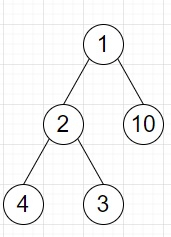# Program to find maximum sum of non-adjacent nodes of a tree in Python

Suppose we have a binary tree, we have to find the maximum sum of the values that can be obtained given no two values can be adjacent parent to child.

So, if the input is likethen the output will be 17 as 10, 4, 3 are not adjacent to each other.

To solve this, we will follow these steps −

• Define a function f() . This will take node
• if node is null, then
• return (0, 0)
• (a, b) := f(left of node)
• (c, d) := f(right of node)
• return a pair (maximum of value of node + b + d and a + c, a + c)
• from the main method call f(root) and return the first value of it

Let us see the following implementation to get better understanding −

## Example

Live Demo

class TreeNode:
def __init__(self, data, left = None, right = None):
self.val = data
self.left = left
self.right = right
def f(node):
if not node:
return 0, 0
a, b = f(node.left)
c, d = f(node.right)
return max(node.val + b + d, a + c), a + c
class Solution:
def solve(self, root):
return f(root)
ob = Solution()
root = TreeNode(1)
root.left = TreeNode(2)
root.right = TreeNode(10) root.left.left = TreeNode(4) root.left.right = TreeNode(3) print(ob.solve(root))

## Input

root = TreeNode(1)
root.left = TreeNode(2)
root.right = TreeNode(10)
root.left.left = TreeNode(4)
root.left.right = TreeNode(3)

## Output

17